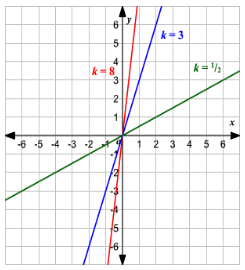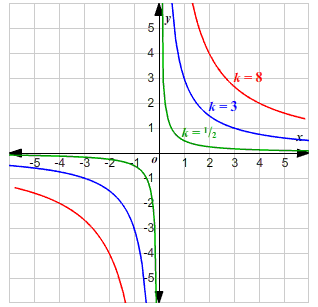# Direct And Inverse Variations

Direct variation means when one quantity changes, the other quantity also changes in direct proportion. Inverse variation is exactly opposite to this. In this article, you will learn the definition of  Direct and inverse variations along with some solved examples.

A few situations in life have either a direct or indirect relationship with each other:

• As the bill at the shopping centre increases, the amount to be paid also increases.
• As the speed of the car decreases, the time taken to reach the destination increases.

If the two values of the objects are related in such a way that an increase or decrease in the value of one object affects the value of the other, which is termed as a variation. The two types of variation are:

## Direct Variation

The quantities are said to be in direct proportion if:

• An increase in the quantity A leads to an increase in quantity B and vice versa, provided their respective ratios are the same.

The methods to solve direct variation problems are tabular and unitary method.

Direct variation is represented as follows:

Y = KX, where K is the constant of proportionality.

The direct variation graph is a line through the origin.## Inverse Variation

The quantities are said to be in inverse proportion if:

• An increase in the quantity A leads to a decrease in quantity B and vice versa.

The graph of inverse variation is as follows:## Direct And Inverse Variation Problems

Example 1: A and B can do a particular work in 72 days. B and C in 120 days. A and C in 90 days. In how many days can A alone do the work?

Solution:

Let us say A, B, C can respectively do work alone in x, y, z days

Therefore, In 1 day A, B, C alone can work in 1 / x, 1 / y, 1 / z days

⇒ (A + B) in 1 day can do 1 / x + 1 / y work

∴ (A + B) can do full work in 1 / ([1 / x] + [1 / y]) day

⇒1 / ([1 / x] + [1 / y]) = 72 i.e.,

1 / x + 1 / y = 1 / 72 ———— (1)

similarly, 1 / y + 1 / z =1 / 120 ———- (2)

1 / z + 1 / x = 1 / 90 ——– (3)

From (1) – (2), 1 / x − 1 / z = 1 / 72 − 1 / 120 ——– (4)

1 / x + 1 / z = 1 / 92 ———– (5)

Adding IV & V, 2 / x = 1 / 72 − 1 / 120 + 1 / 90

= [5 − 3 + 4] /  = 6 / 360 = 1 / 60

∴ x = 120

Example 2: A and B undertake to do a piece of work for Rs. 600. A alone can do it in 6 days, while B alone can do it in 8 days but with the help of C, they finish it in 3 days. Find the share of C.

Solution:

A, B, C can do (alone) work in 6 days, 8 days, & x days (assume) respectively.

∴ Together, they will do it in 1 / ([1 / 6] + [1 / 8] + [1 / x ]) days.

Now, 1 / [(1 / 6) + (1 / 8) + (1 / x)] = 3

⇒ (1 / 6) + (1 / 8) + (1 / x) = 1 / 3

⇒ 1 / x = 1 / 3 − 1 / 6 − 1 / 8 = 1 / 24

⇒ x = 24 days

Efficiency ratio of A : B : C = [1 / 6] : [1 / 8] : [1 / 24] = 4 : 3 : 1

Share of C = (1 / [4 + 3 + 1]) × 600 = Rs 75

Example 3: 45 men can complete a work in 16 days. Six days after they started working, 30 more men joined them. How many days will they now take to complete the remaining work?

Solution:

45 men, 16 days ⇒ 1 work.

1 man, 1 day ⇒ 1 / [45 × 16] work

For the first 6 days: 45 men, 6 days ⇒ [45 × 16] / [45 × 16] work = 3/8 work

Work left = 1 − ⅜ = ⅝

Now, 45 + 30 = 75 men, 1 man, 1 day = 1 / [45 × 16] = work 75 men,

1 day = 75 / [45 × 16] work 75 men, x days = 75x / [45 × 16] work

But = 75x / [45 × 16] work = 5 / 8

⇒ x = 5 / 8 × [(45 × 16) / 75] = 6 days.

Example 4: Two pipes A and B can fill a tank in 24 min. and 32 minutes respectively. If both the pipes are opened together, after how much time should B be closed so that the tank is full in 18 minutes?

Solution:

Let after ‘t’ minutes ‘B’ be closed

For first ‘t’ minutes part filled= t ∗ [1 / 24 + 1 / 32] = t ∗ 7 / 96

Part left = (1 − 7t / 96) ‘A’ filled 1 /24 part in 1 min ⇒ A fills = (1 − [7t / 96]) part in 24

= (1 − 7t / 96) min

Now, [t + 24] (1 − 7t / 96) = 18

⇒t + 24 − [7 t / 4] = 8

⇒6 = 3t / 4

⇒ t = 18

Example 5: Twenty women can do work in sixteen days. Sixteen men can complete the same work in fifteen days. What is the ratio between the capacity of a man and a woman?

Solution:

One woman can do work in 20 × 16 = 320 days

In 1 day, 1 woman can do (1 / 320) part of work

Similarly, 1 man can do work in 16 × 15 = 240 days

∴ In 1 day, one man can do 1 / 240 part of work

Capacity of man: woman = 1 / 240 : 1 / 320 = 1 / 3 : 1 / 4 = 4 : 3

Example 6: A pipe can fill a tank in 15 hours. Due to leak in the bottom, it is filled in 20 hours. If the tank is full, how much time will the leak take to empty it?

Solution:

Filling in 1 hr = [1 / 15] of the tank

Let emptying by leak in 1 hr = [1 / x] tank (where leak empties tank in ‘x’ hrs)

∴ Hours in which it fills along with leak also emptying is

⇒ 1 / ([1 / 15] − [1 / x]) = 20hrs

⇒ [1 / 15] − [1 / x] = [1 / 20]

⇒ [1 / x] =[ 1 / 15] − [1 / 20] = [5 / 30] = [1 / 60]

x = 60 hrs

Example 7: A worker is paid Rs. 139.20 for 3 days.

(i) What will he get in the month of June (in Rs,)?

(ii) For how many days will he be working for Rs. 696?

Solution:

Let Rs. x2 be paid in the month of June.

 Amount paid (x) (in Rs.) x1 = 139.20 x2 No. of days (y) y1 = 3 y2 = 30

Since, it is a case of direct proportion.

∴ [x1 / y1] = [x2 / y2]

⇒ [139.20 / 3] = [x2 / 30]

⇒ x2 = [139.20 × 30] / 3

⇒ x2 = 1392.

Thus, Rs. 1392 will be paid in the month of June.

(ii) Let y2 be the number of days he will work for Rs. 696.

 Amount paid (x) (in Rs.) x1 = 139.20 x2 = 696 No. of days (y) y1 = 3 y2

It is a case of direct proportion.

∴[139.20 / 3] = [696 / y2]

⇒ y2 = [696 × 3 × 10] / 

⇒ y2 = 15

Thus, he will work 15 days for Rs. 696.

Example 8: Which of the following tables shows the inverse proportion?

 (i) x 6 12 30 48 y 250 125 50 31.25 (ii) x 115 130 145 160 y 615 600 585 570 (iii) x 50 100 300 1200 y 300 150 100 75

A)  Only (i)

B)  Both (i) and (ii)

C)  Both (ii) and (iii)

D)  None of these

Solution:

(i) For inverse proportion, xy = constant

Now, 6 × 250 = 1500, 12 × 125 = 1500, 30 × 50 = 1500 and 48 × 31.25 = 1500

∴ The data is in inverse proportion.

(ii) 115 × 615 = 70725, 130 × 600 = 78000

∴ The data is not in inverse proportion.

(iii) 50 × 300 = 15000, 100 × 150 = 15000, and 300 × 100 = 30000

∴ The data is not in inverse proportion.

Example 9: Match the following.

 Column – I Column – II P. x and y are in direct proportion and x = 40 when y = 120. If x = 60 then y = (i) 160 Q. x varies inversely as y and x = 12 when y = 300, if x = 24 then y = (ii) 180 R. x varies directly as y and y = 50 when x = 30, if x = 96 then y = (iii) 130 S. x varies inversely as y and y = 650 when x = 20, if x = 100 then y = (iv) 150

Solution:

P. According to question, [40 / 120] = [60 / y] or y = [60 × 120] / 40 = 180

Q. According to question, [12 × 300] = [24 × y] or y = [12 × 300] /  = 150.

R. According to question, [30 / 50] = [96 / y] or y = [96 × 50] /  = 160

S. According to question, [20 × 650] = [100 × y] or y = [20 × 650] /  = 130